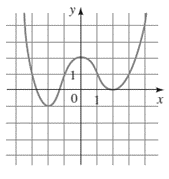# The local maximum and minimum values of the function whose graph is given.### Precalculus: Mathematics for Calcu...

6th Edition
Stewart + 5 others
Publisher: Cengage Learning
ISBN: 9780840068071### Precalculus: Mathematics for Calcu...

6th Edition
Stewart + 5 others
Publisher: Cengage Learning
ISBN: 9780840068071

#### Solutions

Chapter 3.1, Problem 51E
To determine

## To find: The local maximum and minimum values of the function whose graph is given.

Expert Solution

The local maximum value of the function is equal to 2 and the values of local minimum of the function are 1 and 0 .

### Explanation of Solution

Given information:

The graph of the function is:Calculation:

As shown in the graph of the function, the point of local maxima of the function is x=0 and the points of local minima of the function are x=2 and x=2 .

As shown in the graph, the value of the function at x=0 is equal to 2 .

So, the value of local maxima of the function is equal to 2 .

As shown in the graph, the value of the function at x=2 is equal to 1 and the value of the function at x=2 is equal to 0 .

So, the value of local minima of the function are 1 and 0 .

Therefore, the local maximum value of the function is equal to 2 and the values of local minimum of the function are 1 and 0 .

### Have a homework question?

Subscribe to bartleby learn! Ask subject matter experts 30 homework questions each month. Plus, you’ll have access to millions of step-by-step textbook answers!